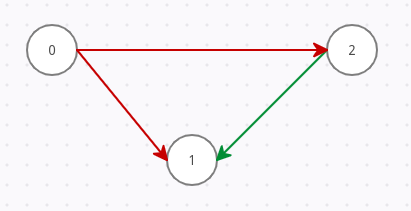2

# Shortest Alternating Path

Difficulty: MEDIUMContributed By
Sounak Majumder
Avg. time to solve
10 min
Success Rate
90%

Problem Statement
Suggest Edit

#### Note:

``````1. This Graph may contain self-loops and parallel edges.

2. The Graph will be directed which means it’s edges are one-directional (from some node u to some node v).

3. Alternating distance is the number of edges between two nodes such that the colours of each consecutive edge in the path are different.

4. If there is no alternating path from a node X to the node with label-0 then print -1 as an answer corresponding to that node.
``````
##### Input format:
``````The first line of the input contains an integer 'T', denoting the number of test cases.

The first line of each test case contains three space-separated integers 'N', 'R' and 'G', denoting the number of nodes, number of Red edges and number of Green edges in the graph respectively.

The next 'R' lines of each test case contain two space-separated integers u and v,  denoting Red coloured directed edges from a node with label u to a node with label v.

The next 'G' lines of each test case contain two space-separated integers u and v,  denoting Green coloured directed edges from a node with label u to the node with label v.
``````
##### Output format:
``````For each test case, print a single line containing 'N' space-separated integers denoting the shortest alternating path length of each node from the node with label 0.

The output of each test case will be printed in a separate line.
``````
##### Note:
``````You do not need to print anything, it has already been taken care of. Just implement the given function.
``````
##### Constraints:
``````1 <= T <= 20
1 <= N, R, G <= 10 ^ 4
0 <= u, v <= N - 1

Time Limit: 1 sec.
``````
##### Sample Input 1:
``````1
3 1 1
0 1
1 2
``````
##### Sample Output 1:
``````0 1 2
``````
##### Explanation of sample input 1:
``````The given graph is as below:
````````````In the given graph, it is clearly visible the alternating path distance of nodes from the 0-labelled node are 0, 1 and 2 respectively.
``````
##### Sample Input 2:
``````1
3 2 1
0 2
0 1
2 1
``````
##### Sample Output 2:
``````0 1 1
``````
##### Explanation of sample input 2:
``````The given graph is as below:
````````````In the given graph, alternating path lengths of nodes with label 0 and 2 are 0 and 1 respectively. But there are two alternative paths that exist for the node with label 1, but we need to consider only the shorter one, hence the shortest alternating path for the node with label-1 is 1.
``````Console